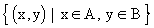Home MonkeyNotes Printable Notes Digital Library Study Guides Study Smart Parents Tips College Planning Test Prep Fun Zone Help / FAQ How to Cite New Title Request

 CHAPTER 6 : RELATIONS, FUNCTIONS AND VARIATION 6.1 Relations Ordered Pairs An ordered pair is a pair of elements, where the order of elements is definite. It is written by enclosing the pair of elements in brackets as ( x, y ). In an ordered pair the two members need not be different. For example ( 7, 7 ). If ( x1 ; y1 ) and ( x2, y2 ) represent ordered pairs then ( x1, y1) = ( x2, y2 ) if and only if x1 = x2 and y1 = y2. But ( 5, 9 ) ╣ ( 9, 5 ). Cartesian product of two set Let A and B be two sets. The cartesian product of two sets A and B is the set of all ordered pairs in which the first element of every pair belongs to the set A and the second belongs to set B. It is written as A ┤ B and read as A cross B. i.e. A ┤ B =Example If A = { 1, 2, 3 }, B = { 4, 5, 6 } then find A ┤ B and B ┤ A Solution : A ┤ B = { ( 1, 4 ), ( 1, 5 ), ( 1, 6 ), ( 2 , 4 ), ( 2, 5 ), ( 2 , 6 ), ( 3, 4 ), ( 3, 5 ), ( 3, 6) } Also B ┤ A = { ( 4, 1 ), ( 4, 2 ), ( 4, 3 ), ( 5, 1 ), ( 5, 2 ), ( 5, 3), ( 6, 1 ), ( 6, 2 ), ( 6, 3 ) } Clearly A ┤ B ╣ B ┤ A Example If A = { x | x Î N Ù x ú 3 } , write A ┤ A and graph it. Solution : A = { x | x Î N Ù x ú 3 } = { 1, 2, 3 } Therefore A ┤ A = { 1, 2, 3 } ┤ { 1, 2, 3 } i.e. A ┤ B = { (1, 1), (1, 2 ), (1, 3), (2, 1), (2, 2), (2, 3), (3, 1), (3, 2), (3,3 ) } Index 6.1 Relations 6.2 Functions6.3 Variation Chapter 7
All Contents Copyright ® All rights reserved.
Further Distribution Is Strictly Prohibited.

 Search: All Products Books Popular Music Classical Music Video DVD Toys & Games Electronics Software Tools & Hardware Outdoor Living Kitchen & Housewares Camera & Photo Cell Phones Keywords: